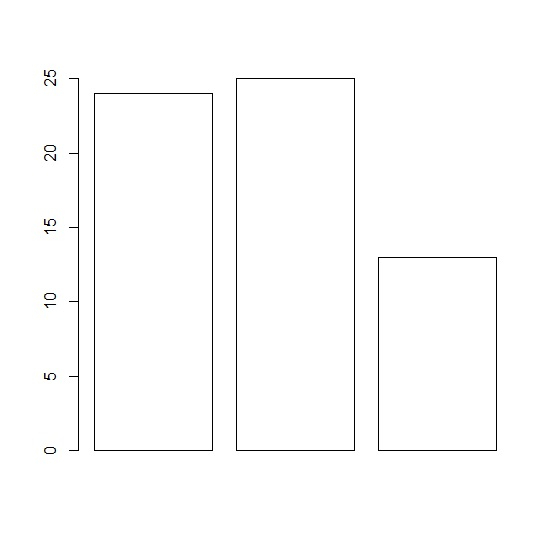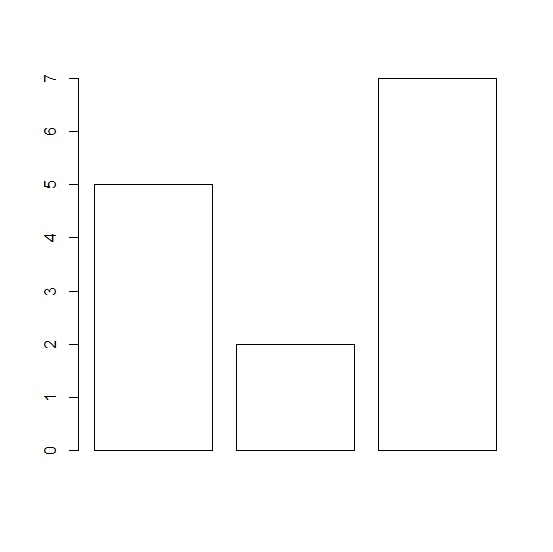# How to create empty bar plot in base R?

To create a bar plot in base R, we can use the function barplot and pass the vector or column of the data frame for which we want to create the bar plot but the bars created by using barplot by default has grey color. Therefore, if we want to create an empty bar plot then setting the color of bars to NA will make the plot an empty bar plot.

## Example1

x<-c(24,25,13)
barplot(x,col = NA)

## Output## Example2

y<-c(5,2,7)
barplot(y,col = NA)

## OutputUpdated on: 06-Mar-2021

235 Views